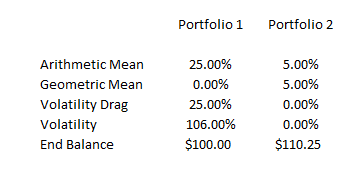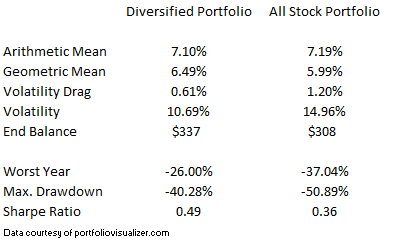# Volatility Can Be Such A Drag| May 20, 2019

Diversification, defensive factor positioning, and managed volatility strategies are all portfolio construction techniques that are often introduced to help control volatility. While risk reduction is a good reason to implement these approaches, it is important to realize that the risk management benefits can actually translate into higher returns. To appreciate this, it is necessary to understand the concept of ‘volatility drag’.

To start, envision an extremely volatile portfolio that doubles in value the first year, but is cut in half the next year. The returns on this portfolio would be 100% for the first year and -50% for the second year. So, to figure out the average annual return (or, arithmetic mean return), we add 100% and -50% and then divide by 2 years, for a result of 25% annually. But how much money did the portfolio actually make? The answer is zero. The portfolio doubled in the first year (say, from \$100 to \$200), but then was cut in half in the second year (from \$200 back to \$100). So, the geometric mean return (which is what we really care about as investors) was 0%. The difference between the arithmetic mean and the geometric mean is due to the volatility of the portfolio and is termed volatility drag. It is the part of the average return that is lost due to movement in the portfolio. In the example above, the portfolio is very volatile so the drag is 25% - the entire amount of the arithmetic mean return.

Now consider a second portfolio that had annual returns of 5% in both years. In this case, both the arithmetic and geometric means would be 5%. Since there is no portfolio volatility, there is no volatility drag. If this portfolio began with \$100 then, after the second year, there would be \$110.25 in the account. This is obviously better than the first portfolio (which has an ending balance of \$100) even though that portfolio can boast of a 25% average annual return!These examples are obviously extremes meant to help illustrate the concepts. Now, let’s look at a real life example that compares a well diversified portfolio with an all stock portfolio from 2000 through the present. The diversified portfolio consists of U.S. large cap stocks (24% of the portfolio), U.S. small cap stocks (12%), international developed market stocks (18%), emerging market stocks (6%), U.S. bonds (30%), and REITs (10%), and is rebalanced once a year to maintain the investment allocations. Look at the results:The arithmetic averages of the two portfolios are very close to one another, but due to lower volatility (and therefore lower volatility drag), the geometric mean of the diversified portfolio is .50% higher on an annualized basis. This may not seem like much, but it adds \$29 to the account balance at the end of the time period which, considering that the portfolio started with \$100, is pretty significant.

The diversified portfolio did have better risk metrics as well, but the point here is that, in addition to helping investors to ‘sleep easy at night’, lowering portfolio volatility adds to geometric returns. As we see, controlling risk can actually add to the bottom line. This is important to consider when evaluating the full benefit of volatility controls within a portfolio’s design. Like many other areas of human interest, in investing, the best offense may be a good defense.

Disclaimer: Diversification, defensive factor positioning, and managed volatility strategies are methods used to help manage investment risk; they do not guarantee a profit or protect against investment loss.